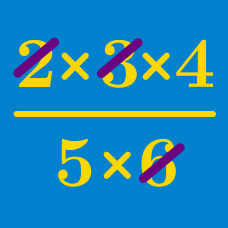Algebra

# Properties Of Arithmetic: Level 1 Challenges

$\large \frac{ \color{#20A900}{2013} \times \color{#3D99F6}{2014} -\color{#20A900}{2013} \times \color{#D61F06}{1392}} { \color{#3D99F6}{2014} - \color{#D61F06}{1392} }= \ \color{#624F41} ?$

$\left ( 1 + \frac{1}{ \color{#D61F06}{4}} \right ) \left ( 1 + \frac{1}{\color{#D61F06}{5}} \right ) \left ( 1 + \frac{1}{\color{#D61F06}{6}} \right ) \left ( 1 + \frac{1}{\color{#D61F06}{7}} \right )$

Evaluate the expression above.

$\Large \sqrt{\color{#69047E}{9999} \times \color{#69047E}{9999}+\color{#20A900}{19999}} = \ \color{fuchsia} ?$

$\large \left (\color{#D61F06}4 + \color{#3D99F6}5 - \color{#20A900}3 \times \color{#69047E}6 \div \color{#624F41}2 \right )^0 + \color{grey}1 = \ ?$

What is $a - (a - b - a - c + a) - b + c?$

×